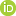This is a Preprint and has not been peer reviewed. This is version 1 of this Preprint.

##### Authors

Frank Male, Jerry L. Jensen

##### Abstract

Reservoir characterization analysis resulting from incorrect applications of statistics can be found in the literature, particularly in applications where integration of various disciplines is needed. Here, we look at three misapplications of ordinary least squares linear regression (LSLR) and show how they can lead to poor results and offer better alternatives, where available. The issues are
1. Application of algebra to an LSLR-derived model to reverse the roles of explanatory and response variables that may give biased predictions. In particular, we examine pore throat size equations (e.g., Winland’s and Pittman’s equations) and find that claims of over-predicted permeability may in part be due to statistical mistakes.
2. Using a log-transformed variable in an LSLR model, de-transforming without accounting for the role of noise gives an equation which under-predicts the mean value. Several approaches exist to address this problem.
3. Mis-application of R2 in three cases that lead to misleading results. For example, model fitting in decline curve analysis gives optimistic R2 values, as is also the case where a multimodal explanatory variable is present.
Using actual and synthetic datasets, we illustrate the effects that these errors have on analysis and some implications for using machine learning results.

##### DOI

https://doi.org/10.31223/osf.io/q7nw6

##### Subjects

Chemical Engineering, Earth Sciences, Engineering, Geology, Petroleum Engineering, Physical Sciences and Mathematics, Statistical Methodology, Statistics and Probability

##### Keywords

data analytics, machine learning, linear regression, petrophysics, Winland equation

##### Dates

Published: 2020-05-11 18:14